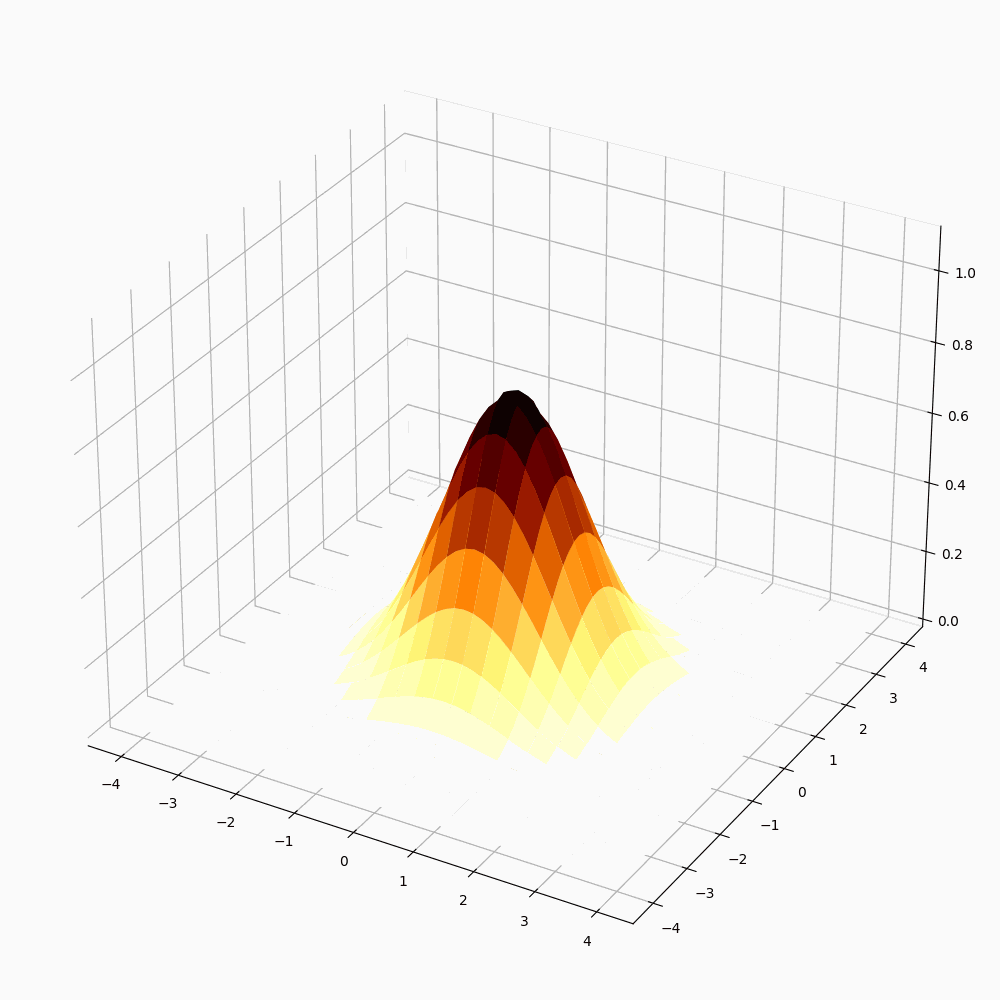# Animate a rotating 3D graph in Matplotlib

To make a rotating 3D graph in matplotlib, we can use Animation class for calling a function repeatedly.

## Steps

• Initialize variables for number of mesh grids, frequency per second to call a function, frame numbers.

• Create x, y, and z array for a curve.

• Make a function to make z array using lambda function.

• To pass a function into the animation class, make a user-defined function to remove the previous plot and plot a surface using x, y, and zarray.

• Create a new figure or activate an existing figure.

• Add a subplot arrangement using subplots() method.

• Set the Z-axis limit using set_zlim() method.

• Call the animation class to animate the surface plot.

• To display the animated plot, use show() method.

## Example

import numpy as np
import matplotlib.pyplot as plt
import matplotlib.animation as animation

plt.rcParams["figure.figsize"] = [7.00, 3.50]
plt.rcParams["figure.autolayout"] = True
N = 50
fps = 250
frn = 75

x = np.linspace(-4, 4, N + 1)
x, y = np.meshgrid(x, x)
zarray = np.zeros((N + 1, N + 1, frn))

f = lambda x, y, sig: 1 / np.sqrt(sig) * np.exp(-(x ** 2 + y ** 2) / sig ** 2)

for i in range(frn):
zarray[:, :, i] = f(x, y, 1.5 + np.sin(i * 2 * np.pi / frn))

def change_plot(frame_number, zarray, plot):
plot.remove()
plot = ax.plot_surface(x, y, zarray[:, :, frame_number], cmap="afmhot_r")

fig = plt.figure()

plot = [ax.plot_surface(x, y, zarray[:, :, 0], color='0.75', rstride=1,
cstride=1)]
ax.set_zlim(0, 1.1)
ani = animation.FuncAnimation(fig, change_plot, frn, fargs=(zarray, plot),
interval=1000 / fps)
ani.save('526.gif')

plt.show()

## OutputUpdated on: 11-May-2021

938 Views